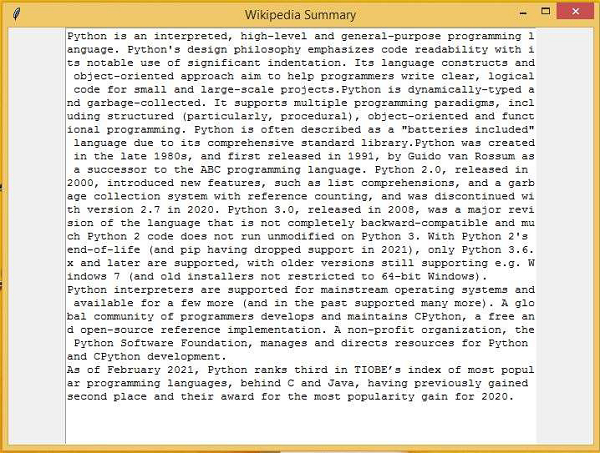# How to Extract Wikipedia Data in Python?

PythonTkinterGUI-Programming

In this article, we will see how to extract Wikipedia data using Python. Python is widely used for creating web scrapers to capture the meta information from the websites.

For this article, we will use the Wikipedia API and library to get the data from the Wikipedia source URL. The API will help to fetch the data from the given URL. Then, we will invoke the method on the given URL and print the information on the screen.

In order to extract data from Wikipedia, we have to first import the wikipedia library in Python using 'pip install wikipedia'.

In this program, we will extract the summary of Python Programming from Wikipedia and print it inside a textbox.

## Example

#Import the tkinter library
from tkinter import *
import tkinter as tk
import wikipedia

win = Tk()
win.geometry("700x500")
win.title("Wikipedia Summary")

result = wikipedia.search("Python Programming")

# get the page Details
page = wikipedia.page(result)

# Get the summary
summary = page.summary

T = tk.Text(win, height=200, width=70)
T.pack()
T.insert(tk.END, summary)

win.mainloop()

## Output

Running the above python code will print the summary related to “Python Programming” sourced from Wikipedia.